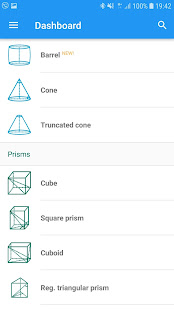Wed, May 25, 2022, 11:33:20 AM Current System Time:

>>

# Geometry PRO v2.32

 Author Page: 1 BannedPM Rank : BeginnerStatus : Member #1Geometry PRO Overview: Geometry PRO is advanced application for solving geometry problems. There is a complete solution delivered for each issue to satisfy every teacher or student. The application solves every algebraic problem including those with: - fractions - roots - powers you can also use parentheses, decimal numbers and Pi number. This application is able to do calculation on the following figures: - square - rectangle - rhombus - parallelogram - triangle - equilateral triangle - right triangle - isosceles triangle - triangle 30-60-90 - circle - annulus - trapezoid - right trapezoid - isosceles trapezoid - Pythagorean theorem - regular hexagon - sphere - cylinder - cone - regular tetrahedron - cube - square prism - cuboid - ellipse - regular Pentagon - Kite - trigonometry - inradius and circumradius of cube - inradius and circumradius on a square or an equilateral triangle - spherical sector - spherical cap - annulus sector PRO version: - Square pyramid - Triangular pyramid - Triangular prism - Regular triangular prism - Thales' theorem - Truncated cone - Regular octagon - Regular dodecagon - Hexagonal prism - Hexagonal pyramid - Pentagonal prism - Barrel - law of sines - law of cosines - spherical wedge - spherical lune An advanced validation of data entry allows you to find the errors quickly and corrects them for you immediately. Geometry PRO will calculate all the parameters of the figure, if you enter the necessary data. The order of data entry depends on you! - Do you want to calculate a side of the square? No problem. Geometry PRO will do it for you. - Do you have an angle and a side of the right triangle? Perfect. Other values can be calculated. None of your geometry tasks will be a problem now with Geometry PRO. This application has very advanced, powerful and easy - to - use interface. Additionally, it contains all the useful formulas which you will need to solve geometry tasks. But thats not enough! You do not have to figure it out how did you get a result. This application not only gives you the solution, it also shows you all the formulas which had been used. Pythagorean Theorem, sines and cosines are no longer a problem.-----------------2 thanks:asfakul20,Quack14, Banned[PM 4017]Rank : Junior MemberStatus : Member #2Nice bro
You are not logged in, please

 Page: 1

Keywords:geometry, overview, advanced, application, solving, problems, complete, solution, delivered, satisfy, teacher, student, solves, algebraic, problem, including,